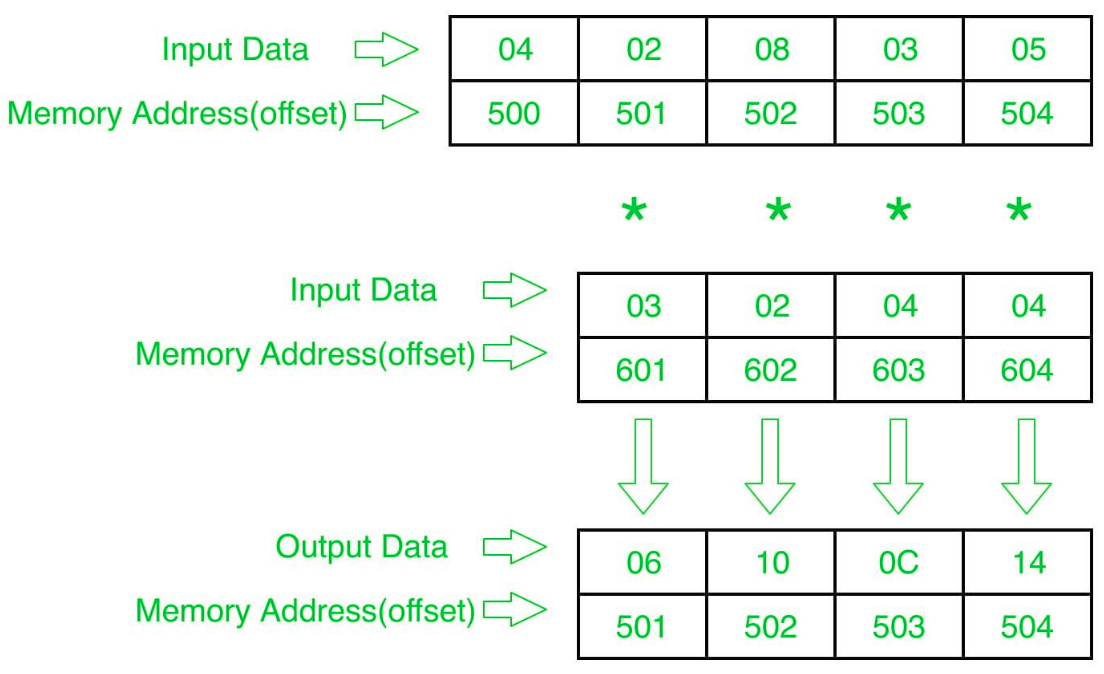# 8086 program to determine product of corresponding elements of two array elements

Problem – Write a program in 8086 microprocessor to find out the product of two arrays of 8-bit n numbers, where size “n” is stored at offset 500 and the numbers of first array are stored from offset 501 and the numbers of second array are stored from offset 601 and store the result numbers into first array i.e offset 501.

Example –Algorithm –

1. Store 500 to SI and 601 to DI and Load data from offset 500 to register CL and set register CH to 00 (for count).
2. Increase the value of SI by 1.
3. Load first number(value) from next offset (i.e 501) to register AL.
4. Multiply the value in register AL by value at offset DI.
5. Store the result (value of register AL ) to memory offset SI.
6. Increase the value of SI by 1.
7. Increase the value of DI by 1.
8. Loop above 5 till register CX gets 0.

Program –

400 MOV SI, 500 SI<-500
403 MOV CL, [SI] CL<-[SI]
405 MOV CH, 00 CH<-00
407 INC SI SI<-SI+1
408 MOV DI, 601 DI<-601
40B MOV AL, [SI] AL<-[SI]
40D MUL [DI] AX=AL*[DI]
40F MOV [SI], AL AL->[SI]
411 INC SI SI<-SI+1
412 INC DI DI<-DI+1
415 HLT end

Explanation –

1. MOV SI, 500: set the value of SI to 500
2. MOV CL, [SI]: load data from offset SI to register CL
3. MOV CH, 00: set value of register CH to 00
4. INC SI: increase value of SI by 1.
5. MOV DI, 600: set the value of DI to 600.
6. MOV AL, [SI]: load value from offset SI to register AL
7. MUL AL, [DI]: multiply value of register AL by content at offset DI.
8. MOV [SI], AL: store value of register AL at offset SI.
9. INC SI: increase value of SI by 1.
10. INC DI: increase value of DI by 1.